## 17.2.1 Nodes and node positions

There are two basic kinds of satellite nodes: geostationary and non-geostationary satellite nodes. In addition, terminal nodes can be placed on the Earth's surface. As is explained later in Section 17.3, each of these three different types of nodes is actually implemented with the same class SatNode object, but with different position, handoff manager, and link objects attached. The position object keeps track of the satellite node's location in the coordinate system as a function of the elapsed simulation time. This position information is used to determine link propagation delays and appropriate times for link handoffs. Section 5.3 introduced the "node-config" utility used to prime the node generator for different types of satellite nodes.

Figure 17.2 illustrates the spherical coordinate system, and the corresponding Cartesian coordinate system. The coordinate system is centered at the Earth's center, and the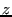axis coincides with the Earth's axis of rotation.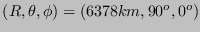corresponds to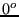longitude (prime meridian) on the equator.

Specifically, there is one class of satellite node Class Node/SatNode, to which one of three types of Position objects may be attached. Each SatNode and Position object is a split OTcl/C++ object, but most of the code resides in C++. The following types of position objects exist:

• Position/Sat/Term A terminal is specified by its latitude and longitude. Latitude ranges fromand longitude ranges from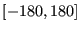, with negative values corresponding to south and west, respectively. As simulation time evolves, the terminals move along with the Earth's surface. The node generator can be used to create a terminal with an attached position object as follows:
$ns node-config -satNodeType terminal \bs (other node config commands go here...) set n1 [$ns node]
$n1 set-position$lat $lon; # in decimal degrees  • Position/Sat/Geo A geostationary satellite is specified by its longitude above the equator. As simulation time evolves, the geostationary satellite moves through the coordinate system with the same orbital period as that of the Earth's rotation. The longitude ranges fromdegrees. As we describe further below, two flavors of geostationary nodes exist: geo'' (for processing satellites) and geo-repeater'' (for bent-pipe satellites). The node generator can be used to create a geostationary satellite with an attached position object as follows: $ns node-config -satNodeType geo (or geo-repeater'') \bs
(other node config commands go here...)
set n1 [$ns node]$n1 set-position $lon; # in decimal degrees  • Position/Sat/Polar A polar orbiting satellite has a purely circular orbit along a fixed plane in the coordinate system; the Earth rotates underneath this orbital plane, so there is both an east-west and a north-south component to the track of a polar satellite's footprint on the Earth's surface. Strictly speaking, the polar position object can be used to model the movement of any circular orbit in a fixed plane; we use the term polar'' here because we later use such satellites to model polar-orbiting constellations. Satellite orbits are usually specified by six parameters: altitude, semi-major axis, eccentricity, right ascension of ascending node, inclination, and time of perigee passage. The polar orbiting satellites in ns have purely circular orbits, so we simplify the specification of the orbits to include only three parameters: altitude, inclination, and longitude, with a fourth parameter alpha specifying initial position of the satellite in the orbit, as described below. Altitude is specified in kilometers above the Earth's surface, and inclination can range fromdegrees, with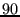corresponding to pure polar orbits and angles greater thandegrees corresponding to retrograde'' orbits. The ascending node refers to the point where the footprint of the satellite orbital track crosses the equator moving from south to north. In this simulation model, the parameter longitude of ascending node specifies the earth-centric longitude at which the satellite's nadir point crosses the equator moving south to north.17.3 Longitude of ascending node can range fromdegrees. The fourth parameter, alpha, specifies the initial position of the satellite along this orbit, starting from the ascending node. For example, an alpha of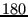degrees indicates that the satellite is initially above the equator moving from north to south. Alpha can range fromdegrees. Finally, a fifth parameter, plane, is specified when creating polar satellite nodes- all satellites in the same plane are given the same plane index. The node generator used to create a polar satellite with an attached position object as follows: $ns node-config -satNodeType polar \bs
(other node config commands go here...)
set n1 [$ns node]$n1 set-position $alt$inc $lon$alpha \$plane


Tom Henderson 2011-11-05